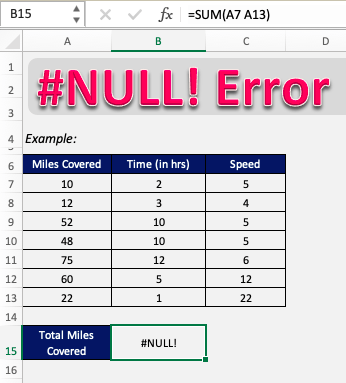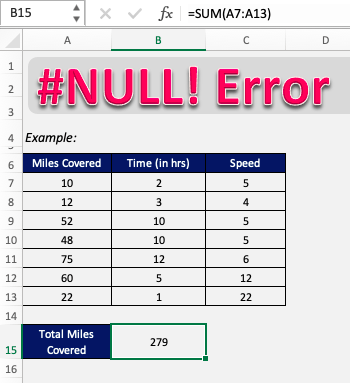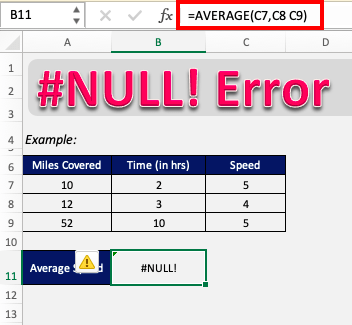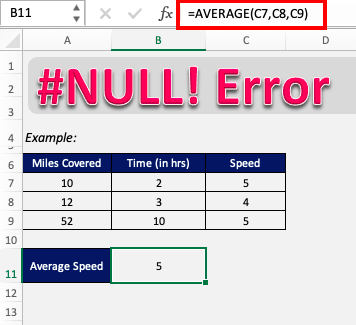Null value in Excel is an uncommon error that occurs when there is something fundamentally wrong with the formula. If it occurs in Excel, it can be confusing to some people, but it really is very simple to correct.

### What is NULL value in Excel?

NULL in Excel occurs simply when the range provided in the formula is not valid and there is a typo in the formula. This can be mainly due to two reasons :

• Wrong input instead of a colon (:)
• Wrong input instead of a comma (,)

In most cases, this wrong input is that users insert a space rather than the appropriate character. Simply correcting these typos can remove the error. Let’s look into this in more detail.

Follow this detailed tutorial on null Excel and download this Excel workbook to practice along understand better:

### How to fix NULL value in Excel?

Example 1 – Wrong input instead of a colon (:)

Sometimes in a formula, where there is supposed to be “:”(colon), you might have entered space. This can be the result of the error that we are getting as shown below.In the formula bar, we can see that instead of =SUM(A7:A13), it is entered as =SUM(A7 A13), which is the cause of the error.

So to correct this, we have to replace that space with a colon(:).Like so! As the typo has been corrected, the error has been removed and the formula is returning the correct value.

Example 2 – Wrong input instead of a comma (,)

Sometimes in a formula, where there is supposed to be “,”(comma), you might have entered space.

In this example, we are trying to calculate the average speed in cell B11 based on the 3 entries mentioned in rows 7, 8, and 9. The formula used is:

=AVERAGE(C7,C8 C9)

This can lead to an error that we are getting as shown below:Here, instead of =AVERAGE(C7,C8 C9) you should enter the formula as =AVERAGE(C7,C8,C9).

There should have been a comma between C8 and C9 but instead, there has been a typing error and instead of the comma, space was inserted.

Simply removing the space and adding the comma rectifies this error.### Conclusion

So now it should be clear that the NULL value is a simple but uncommon error that occurs due to a typing error, making the inputs of a formula invalid or if the separators of different arguments in a formula are entered incorrectly.

It doesn’t necessarily have to be a space, but can be any incorrect character. Now that you have understood the fundamentals of Excel null value, you too can easily overcome it!

Click here to learn about the Top 20 Common Errors that you may encounter while working on Excel.